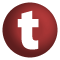# Equiv

#### trait Equiv[T] extends AnyRef

A trait for representing equivalence relations. It is important to distinguish between a type that can be compared for equality or equivalence and a representation of equivalence on some type. This trait is for representing the latter.

An equivalence relation is a binary relation on a type. This relation is exposed as the `equiv` method of the `Equiv` trait. This relation must be:

• reflexive: `equiv(x, x) == true`, for any `x` of type `T`.
• symmetric: `equiv(x, y) == equiv(y, x)`, for any `x` and `y` of type `T`.
• transitive: if `equiv(x, y) == true` and `equiv(y, z) == true` then `equiv(x, z) == true`, for any `x`, `y`, and `z` of type `T`.

known subclasses: PartialOrdering

1. AnyRef
2. Any

### Value Members

1. #### def equals(arg0: Any): Boolean

This method is used to compare the receiver object (`this`) with the argument object (`arg0`) for equivalence

This method is used to compare the receiver object (`this`) with the argument object (`arg0`) for equivalence.

The default implementations of this method is an equivalence relation:

• It is reflexive: for any instance `x` of type `Any`, `x.equals(x)` should return `true`.
• It is symmetric: for any instances `x` and `y` of type `Any`, `x.equals(y)` should return `true` if and only if `y.equals(x)` returns `true`.
• It is transitive: for any instances `x`, `y`, and `z` of type `AnyRef` if `x.equals(y)` returns `true` and `y.equals(z)` returns `true`, then `x.equals(z)` should return `true`.

If you override this method, you should verify that your implementation remains an equivalence relation. Additionally, when overriding this method it is often necessary to override `hashCode` to ensure that objects that are "equal" (`o1.equals(o2)` returns `true`) hash to the same `Int` (`o1.hashCode.equals(o2.hashCode)`).

arg0

the object to compare against this object for equality.

returns

`true` if the receiver object is equivalent to the argument; `false` otherwise.

definition classes: AnyRef ⇐ Any
2. #### def equiv(x: T, y: T): Boolean

Returns `true` iff `x` is equivalent to `y`

Returns `true` iff `x` is equivalent to `y`.

attributes: abstract
3. #### def hashCode(): Int

Returns a hash code value for the object

Returns a hash code value for the object.

The default hashing algorithm is platform dependent.

Note that it is allowed for two objects to have identical hash codes (`o1.hashCode.equals(o2.hashCode)`) yet not be equal (`o1.equals(o2)` returns `false`). A degenerate implementation could always return `0`. However, it is required that if two objects are equal (`o1.equals(o2)` returns `true`) that they have identical hash codes (`o1.hashCode.equals(o2.hashCode)`). Therefore, when overriding this method, be sure to verify that the behavior is consistent with the `equals` method.

definition classes: AnyRef ⇐ Any
4. #### def toString(): String

Returns a string representation of the object

Returns a string representation of the object.

The default representation is platform dependent.

definition classes: AnyRef ⇐ Any